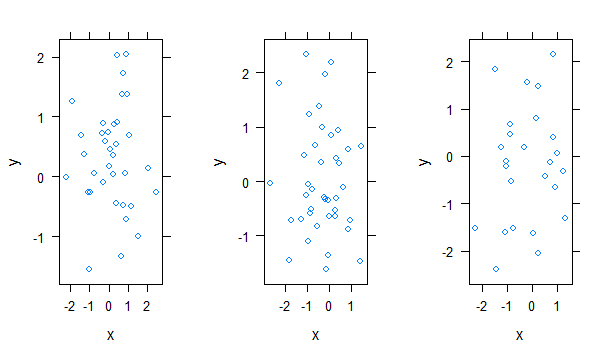# Draw Multiple lattice Plots in One Window in R

• Last Updated : 06 Jun, 2021

In this article, we will discuss how to draw multiple lattice plots in One window.

### Module Used

• lattice: The lattice package uses grid package to provide better relationships between the data. It is an add-on package for the implementation of Trellis graphics (graphics that shows relationships between variables conditioned together).
• gridExtra: This package provides multiple functions that define various grids to be used to arrange multiple plots.

Both of these packages have to be installed on the system explicitly.  To begin with, we need to set up a dataframe, that contains data to be plotted on the x and y-axis and also another column that can be used to differentiate between lattices. For each unique value of this column, a different lattice plot will be generated.

Individual lattice plots are created via xyplot() function, this function produces a scatter plot. For each row, a certain condition decides in which lattice it should be placed.

Syntax:

xyplot(formula, data, ..)

Parameter:

• formula: To specify certain condition
• data: dataframe to be plotted

Once all the lattice plots are ready it is time to arrange them in one window for this arrange() function of the gridExtra package is employed.

arrange() function in R Language is used for reordering of table rows with the help of column names as expression passed to the function.

Syntax: arrange(x, expr)

Parameters:
x: data set to be reordered
expr: logical expression with column name

Example:

## R

 `library``(lattice)``library``(gridExtra)`` ` `df<-``data.frame``(x = ``rnorm``(100),``               ``y = ``rnorm``(100),``               ``z = ``c``(``rep``(``"A"``, 35),``                     ``rep``(``"B"``, 40),``                     ``rep``(``"C"``, 25))``)``                ` `df`` ` `lat1 <- ``xyplot``(y~x,df[df\$z == ``'A'``,])``lat2 <- ``xyplot``(y~x,df[df\$z == ``'B'``,])``lat3 <- ``xyplot``(y~x,df[df\$z == ``'C'``,])`` ` `grid.arrange``(lat1, lat2, lat3, ncol = 3)`

Output:My Personal Notes arrow_drop_up